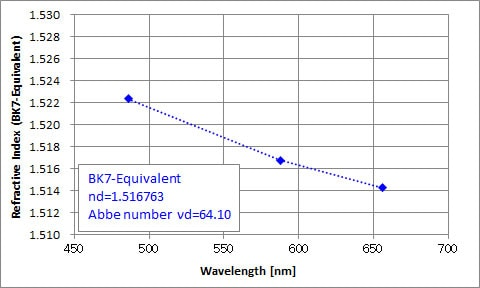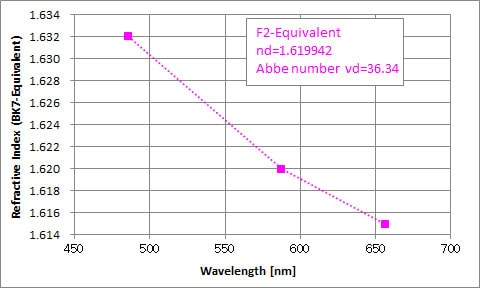# Refractive index and dispersion of optical glass

### Summary

The KPR-300 measures the refractive index automatically at multiple wavelengths (d-line, C-line, F-line), and calculates Abbe numbers. The measuring method of this instrument is a V-block method (Vee-block or Hilger-Chance method) with a reputation for refractive index measurement of optical glass and resin. Therefore, it can measure the refractive index with excellent accuracy and reproducibility.
Even with optical glass for polishing with stable optical characteristics, the general tolerance is ± 0.0003 in refractive index and ± 0.5% in Abbe number. In order to ensure stable optical performance taking into account the process of annealing, management is important depending on measured values.
It can be used for material development, quality control at the time of material manufacturing, and acquisition of necessary information to design optimized for use conditions.

Related information: Refractive index and dispersion of small diameter lens

### Measurement example

 Samples : Crown glass slab(Equivalent to BK7), Flint glass slab (Equivalent to F2)
 Conditions : Measurement temperature - 23 ℃ Measurement wavelength - 3 wavelength (F-line, d-line, C-line) Contact liquid – nd 1.52 (@ BK7), nd 1.62 (@ F2)
 Result : At crown glass (BK7-equivalent), results of refractive index nd 1.516763 and Abbe number νd 64.10 were obtained. At flint glass (F2-equivalent), results of refractive index nd 1.619942 and Abbe number νd 36.34 were obtained. The refractive index can be measured with the resolution (6 digits below the decimal point) necessary for grasping the optical performance, and the Abbe number can be calculated.Fig1. Refractive index measurement result of crown glass(Equivalent to BK7)Fig2. Refractive index measurement result of flint glass(Equivalent to F2)

※It is a typical value obtained by this measurement. It does not guarantee the refractive index and the Abbe number of each sample.

No Item Note